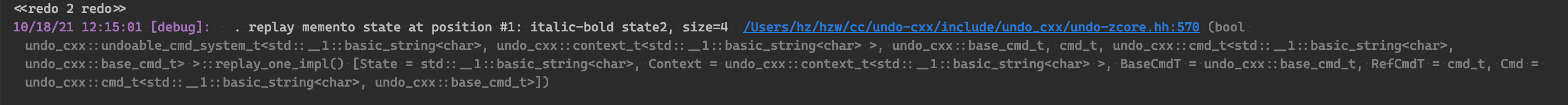## `std::declval` 和 `decltype`

### 关于 decltype

`decltype(expr)` 是一个 C++11 新增的关键字，它的作用是将实体或者表达式的类型求出来。

``````
1
2
3
4
5
6

#include <iostream>
int main() {
int i = 33;
decltype(i) j = i * 2;
std::cout << j;
}

````````````
1
2
3
4
5
6
7
8
9
10

void test_state_meta() {
using M = decltype(m);
// equals to: using M = machine_t<my_state, void, payload_t<my_state>>;

// @formatter:off
// states
m.state().set(my_state::Initial).as_initial().build();
// ...
}

``````

### 关于 `std::declval`

`std::declval<T>()` 也没什么好说的，它能返回类型 T 的右值引用参考。

``````
1
2

T obj{};
T &objref = obj{};

``````

``````
1
2
3
4
5
6
7
8
9
10
11
12
13
14
15
16
17
18
19
20
21
22

#include <iostream>

namespace {
struct base_t { virtual ~base_t(){} };

template<class T>
struct Base : public base_t {
virtual T t() = 0;
};

template<class T>
struct A : public Base<T> {
~A(){}
virtual T t() override { std::cout << "A" << '\n'; return T{}; }
};
}

int main() {
decltype(std::declval<A<int>>().t()) a{}; // = int a;
decltype(std::declval<Base<int>>().t()) b{}; // = int b;
std::cout << a << ',' << b << '\n';
}

``````

#### declval 的力量

`declval(expr)` 的核心力量在上面的例子中显示的很明白：它不会对 expr 真正求值。所以你不必在 expr 处产生任何临时对象，也不会因为表达式很复杂而发生真实的计算。这对于元编程的复杂环境是非常有用的。FROM: HERE

##### 无默认构造函数

``````
1
2
3
4
5
6
7
8

struct A{
A() = delete;
int t(){ return 1; }
}

int main(){
}

``````

``````
1
2
3

int main(){
decltype(std::declval<A>().t()) i; // OK
}

``````

## Tricks

### 采用一个普通的抽象类作为基类

``````
1
2
3
4
5
6
7
8
9
10
11
12
13
14
15
16
17
18
19
20
21
22
23
24

struct base {
virtual ~base_t(){}

void operation() { do_sth(); }

protected:
virtual void do_sth() = 0;
};

template <class T>
struct base_t: public base{
protected:
virtual void another() = 0;
};

template <class T, class C=std::list<T>>
struct vec_style: public base_t<T> {
protected:
void do_sth() override {}
void another() override {}

private:
C _container{};
};

``````

### 纯虚类如何放入容器里

``````
1
2
3
4
5
6
7
8
9
10
11
12
13
14
15
16
17
18
19
20
21
22
23
24
25

#include <iostream>

namespace {
struct base {};

template<class T>
struct base_t : public base {
virtual ~base_t(){}
virtual T t() = 0;
};

template<class T>
struct A : public base_t<T> {
A(){}
A(T const& t_): _t(t_) {}
~A(){}
T _t{};
virtual T t() override { std::cout << _t << '\n'; return _t; }
};
}

int main() {
}

``````

``````
1
2
3
4
5

std::vector<std::shared_ptr<base_t<int>>> vec;

int main(){
vec.push_back(std::make_shared<A<int>>(1));
}

``````

### Runtime Polymorphism

``````
1
2
3
4
5
6
7
8
9
10
11
12
13
14
15
16
17
18
19
20
21
22
23
24
25
26
27
28
29
30
31
32
33
34
35
36
37
38
39
40
41
42
43
44
45
46
47
48
49
50
51
52
53

#include <iostream>
#include <memory>
#include <string>
#include <vector>

class Animal {
public:
struct Interface {
virtual std::string toString() const = 0;
virtual ~Interface()                 = default;
};
std::shared_ptr<const Interface> _p;

public:
Animal(Interface* p) : _p(p) { }
std::string toString() const { return _p->toString(); }
};

class Bird : public Animal::Interface {
private:
std::string _name;
bool        _canFly;

public:
Bird(std::string name, bool canFly = true) : _name(name), _canFly(canFly) {}
std::string toString() const override { return "I am a bird"; }
};

class Insect : public Animal::Interface {
private:
std::string _name;
int         _numberOfLegs;

public:
Insect(std::string name, int numberOfLegs)
: _name(name), _numberOfLegs(numberOfLegs) {}
std::string toString() const override { return "I am an insect."; }
};

int main() {
std::vector<Animal> creatures;

creatures.emplace_back(new Bird("duck", true));
creatures.emplace_back(new Bird("penguin", false));
creatures.emplace_back(new Insect("spider", 8));
creatures.emplace_back(new Insect("centipede", 44));

// now iterate through the creatures and call their toString()

for (int i = 0; i < creatures.size(); i++) {
std::cout << creatures[i].toString() << '\n';
}
}

``````

## 后记

`std::declval<T>()` 也被典型地用在编译期测试等用途，下一次有闲再做探讨吧，那个话题太大了。